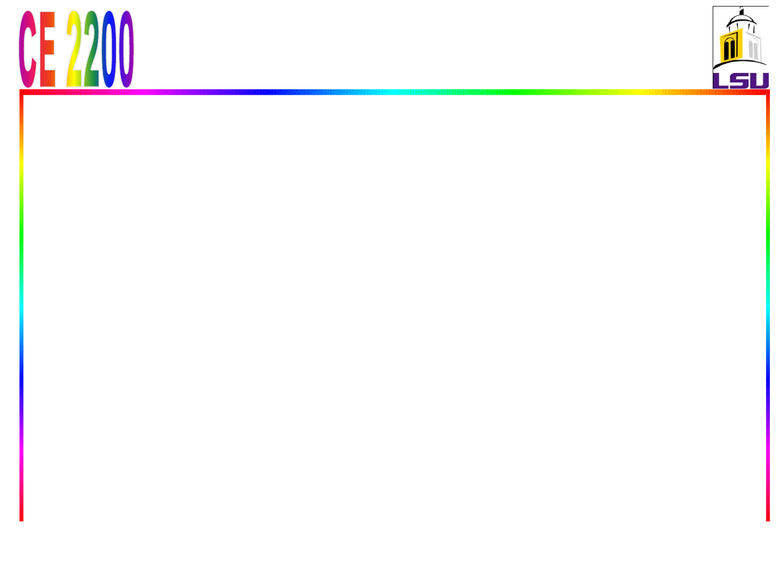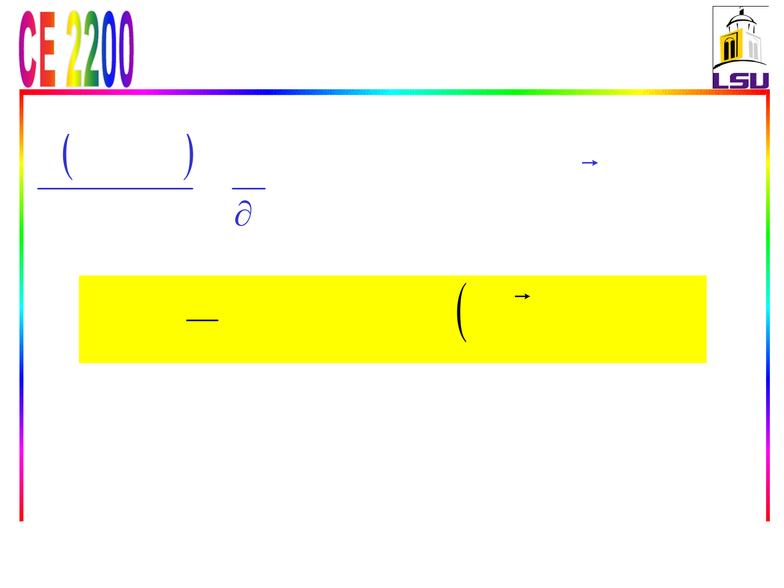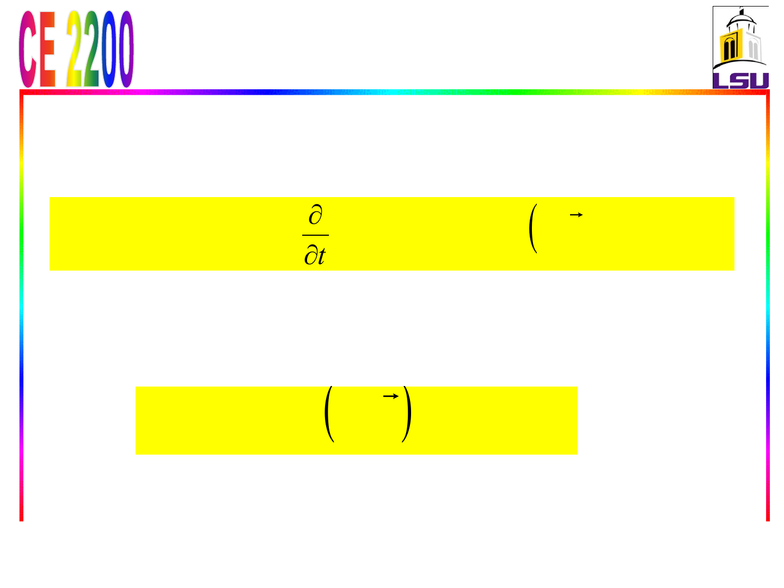Class Notes (1,100,000)
US (480,000)
LSU (7,000)
CE (60)
All (8)
Lecture

# Lecture1415

Department
Civil Engineering
Course Code
CE 2200
Professor
All

This preview shows pages 1-3. to view the full 46 pages of the document.10/ 20/ 2014 1
Lectures-14 & 15: Chapter 6
Momentum Equation
Objectives: Use control volume analysis to
determine forces associated with fluid flow.
Outline
Derivation of Momentum Equation
Interpretation of the Momentum Equation
Procedures for Using Momentum Equation
Examples
Navier-Stokes Equations
Summary

Only pages 1-3 are available for preview. Some parts have been intentionally blurred.10/ 20/ 2014
2
6-1: Linear Momentum
Equation: Derivation
Reynolds transport theorem (by setting B = ___ and b = __)
Linear momentum equation
Here Vr= _______is the fluid velocity relative to the control
surface (for use in mass flow rate calculations at all locations
where the fluid crosses the CS), and Vis the fluid velocity as
viewed from an inertial reference frame. The product
(Vr.n)dA represents the mass flow rate through area
element dA into or out of the CV.
CSCV dnd
tdt
dAVVV
Mom
r
sys
(1a) dAnVVdV
t
FCV CS r


Only pages 1-3 are available for preview. Some parts have been intentionally blurred.10/ 20/ 2014 3
Linear Momentum Equation:
Derivation: Special Cases
For a fixed control volume (no motion or deformation of
control volume), Vr= _and the linear momentum equation
becomes
Note that the momentum equation is ______ equation, and
thus each term should be treated as a vector.
Most momentum problems considered in this text are
(1b) :CV Fixed dAnVVdV
t
FCV CS

(1c) dAnVVF CS r